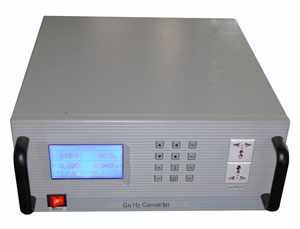Categories

# Hertz (Hz)

## What is Hertz?

Hertz, in short Hz, is the basic unit of frequency, to commemorate the discovery of electromagnetic waves by the German physicist Heinrich Rudolf Hertz. In 1888, German physicist Heinrich Rudolf Hertz (Feb-22, 1857 to Jan-1, 1894), the first person confirmed the existence of radio waves, and had a great contribution in Electromagnetism, so the SI unit of frequency Hertz is named as his name.## What is Hz used for?

Hz (Hertz) is the frequency unit of the vibration cycle time of electric, magnetic, acoustic and mechanical vibration, i.e. the number of times per second (cycle/sec).

1 Hertz means one vibration cycle per second, 50 Hertz means 50 vibration cycles per second while 60 Hertz means 60 vibration cycles per second. Hz is a very small unit, usually coupled with kHz (kilohertz), MHz (Megahertz), GHz (Gigahertz) etc.

kHz is frequency unit of alternating current (AC) or electromagnetic wave (EM), equal to 1000 hertz (1000 Hz). This unit is also used for measuring and describing the signal bandwidth.

1 kHz frequency AC signal is within the human auditory sensation area. The EM wavelength of 1 kHz signal is 300 km, which is about 190 miles. Standard amplitude modulation (AM) broadcast bandwidth is in the range of 535 kHz to 1605 kHz. Some EM transmissions are in millions of kHz.

kHz is a relative small unit of frequency, more common units are MHz, equal to 1,000,000 Hz or 1,000 kHz, and GHz, which equal to 1,000,000,000 Hz or 1,000,000 kHz.

Hz Common Values
For sounds, the human hearing range is 20 Hz ~ 20000 Hz, lower than this range is called infrasound, higher than this range is called ultrasound.
ITU defines radio frequency range:1. Ultra Low Frequency (ULF): 3 ~ 30 kilohertz (kHz)
2. Low Frequency (LF): 30 ~ 300 kilohertz (kHz)
3. Intermediate Frequency (MF): 300 ~ 3000 kilohertz (kHz)
4. High Frequency (HF): 3 ~ 30 megahertz (MHz)
5. Very High Frequency (VHF): 30 ~ 300 megahertz (MHz)
6. Ultra High Frequency (UHF): 300 ~ 3000 megahertz (MHz)
7. Super High Frequency (SHF): 3 ~ 30 GHz (GHz)
8. Extremely High Frequency (EHF): 30 ~ 300 GHz (GHz)

## What is Hz Converter?

A Hz Converter is an electronic device to convert mains power (50 Hz, 60 Hz, etc.) to variable Hertz, variable Volts for home/industry appliances compatibility. It's different with a variable frequency drive which is only for AC motors due the output waveform is square wave, and output Hertz and Volts can not be changed in separate. A Hz converter outputs pure sine wave, Hz and Volts can be adjusted in separate, e.g. 50 Hz 220V, 50 Hz 400V, 60 Hz 110V, 60 Hz 480V, 400 Hz 115V, 230V, 240V etc. with random combination for different equipment running on its perfect condition. By using a Hz converter, you can even go for much higher frequencies, like 120 Hz, 400 Hz for aircrafts, ships, military utilities etc.

Can a 50 Hertz motor run on 60 Hertz power system?
Since the formula for controlling the synchronous speed of a three phase motor is = [(120* Hz) / Motor Poles] if this is a 4-pole motor then at 50 Hz the speed would be 1500 RPM whereas at 60 Hz the speed would be 1800 RPM. Since motors are constant torque machines then by applying the formula that HP = (torque*RPM)/5252 then you can see that with a 20% increase in speed the motor would also then be able to produce 20% more horsepower. The motor would be able to produce rated torque at both frequencies only apply if the V/Hz ratio is constant, meaning that at 50 Hz the supply voltage would need to be 380V and at 60 Hz the supply voltage would need to be 460V. In both cases the V/Hz ratio is 7.6V/Hz.